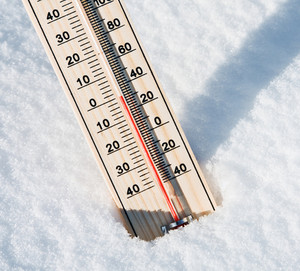## Problem 2: How's the Weather?"Ugh, It's been so hot out lately, why does it have to be 32 degrees…"
"32 degrees? That's freezing!"

You're had quite enough of confusing conversations with Americans who assume you're using Farenheit when Celsius is clearly the way to go. Rather than argue with them, you've settled for writing a program to conveniently convert temperatures measured in degrees Celsius to Farenheit instead.

You came across the following formula, which holds true given that F is a temperature in degrees Farenheit while C is that same temperature in degrees Celsius:

C = 5/9 × (F − 32)

Given a value of C, which is an integer between −40 and 40 (inclusive), determine the corresponding value of F, so that you can express the equivalent temperature in degrees Farenheit for the Americans' benefit.

It's guaranteed that C will be chosen such that F will come out to exactly an integer, but you may output it with 0 or more digits after the decimal point.

### Input Format

The first and only line of input consists of a single integer, C.

### Output Format

Output a single integer, the temperature in degrees Farenheit which is equivalent to C degrees Celsius.

```20
```

### Sample Output

```68
```

Point Value: 3
Time Limit: 2.00s
Memory Limit: 16M